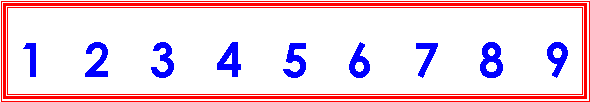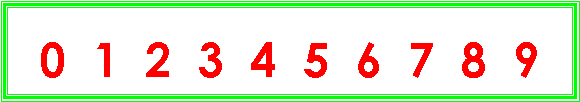#### You may also like### Have You Got It?

Can you explain the strategy for winning this game with any target?### Counting Factors

Is there an efficient way to work out how many factors a large number has?### Summing Consecutive Numbers

15 = 7 + 8 and 10 = 1 + 2 + 3 + 4. Can you say which numbers can be expressed as the sum of two or more consecutive integers?

# Adding All Nine

##### Age 11 to 14Challenge Level

Make a set of numbers that use all the digits from $1$ to $9$, once and once only.For instance, we could choose:

$638, 92, 571$ and $4$

$638 + 92 + 571 + 4 = 1305$

$1305$ is divisible by $9$ (it is $145\times 9$)

(use a calculator to check this if you do not know yet how to divide by $9$)

Add each of the digits in the number $1305$ . What is their sum?

Or, perhaps we could choose:

$921, 4357$ and $68$

$921 + 4357 + 68 = 5346$

$5346$ is divisible by $9$ (it is $594 \times 9$)

Now try some other possibilities for yourself!

I wonder what happens if we use all $10$ digits from $0$ to $9$, once and once only?Try some for yourself!

What do you think would happen if we used the eight digits from $1$ to $8$?

Test your hypothesis by trying some possibilities for yourself!

Were you correct?

Is there a pattern beginning to emerge? Do you have theory that might explain what is happening?

Try some different sets of digits for yourself!